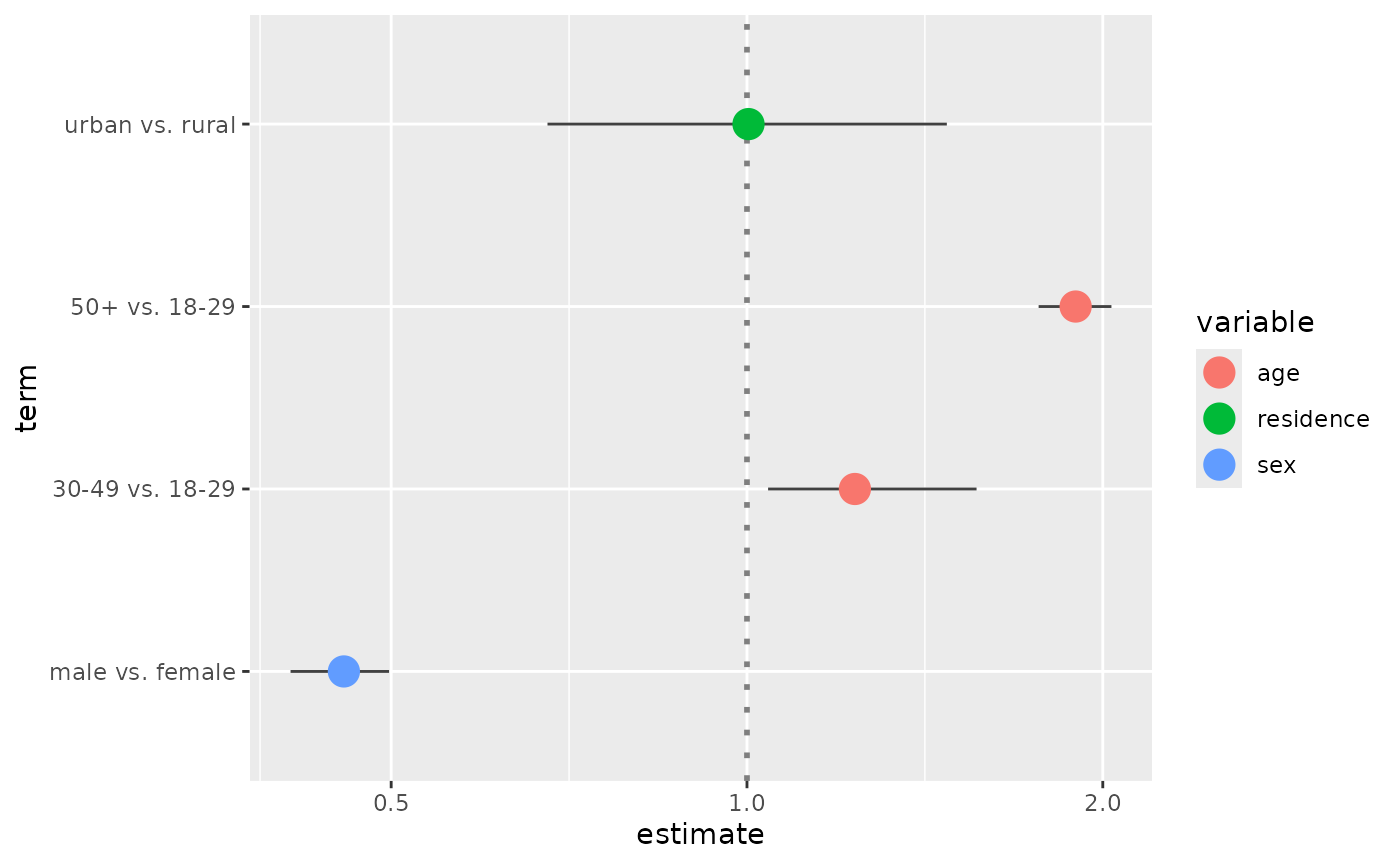library(GGally)
#> Registered S3 method overwritten by 'GGally':
#>   method from
#>   +.gg   ggplot2

# GGally::ggcoef()

The purpose of this function is to quickly plot the coefficients of a model. For an updated and improved version, see GGally::ggcoef_model() and the corresponding vignette.

## Quick coefficients plot

To work automatically, this function requires the broom package. Simply call ggcoef() with a model object. It could be the result of stats::lm, stats::glm or any other model covered by broom and its broom::tidy method1.

reg <- lm(Sepal.Length ~ Sepal.Width + Petal.Length + Petal.Width, data = iris)
ggcoef(reg)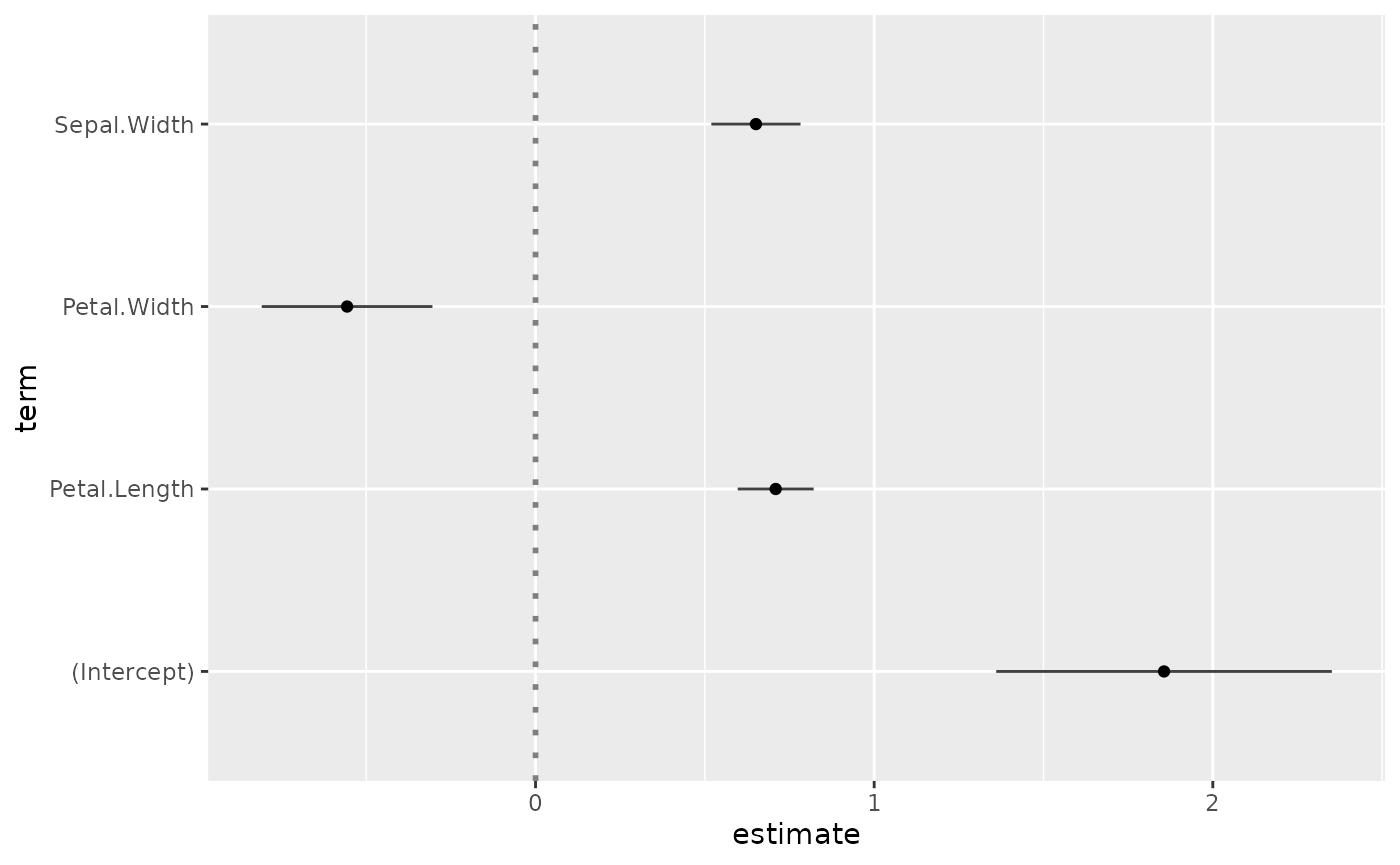In the case of a logistic regression (or any other model for which coefficients are usually exponentiated), simply indicated exponentiate = TRUE. Note that a logarithmic scale will be used for the x-axis.

d <- as.data.frame(Titanic)
log.reg <- glm(Survived ~ Sex + Age + Class, family = binomial, data = d, weights = d$Freq) ggcoef(log.reg, exponentiate = TRUE)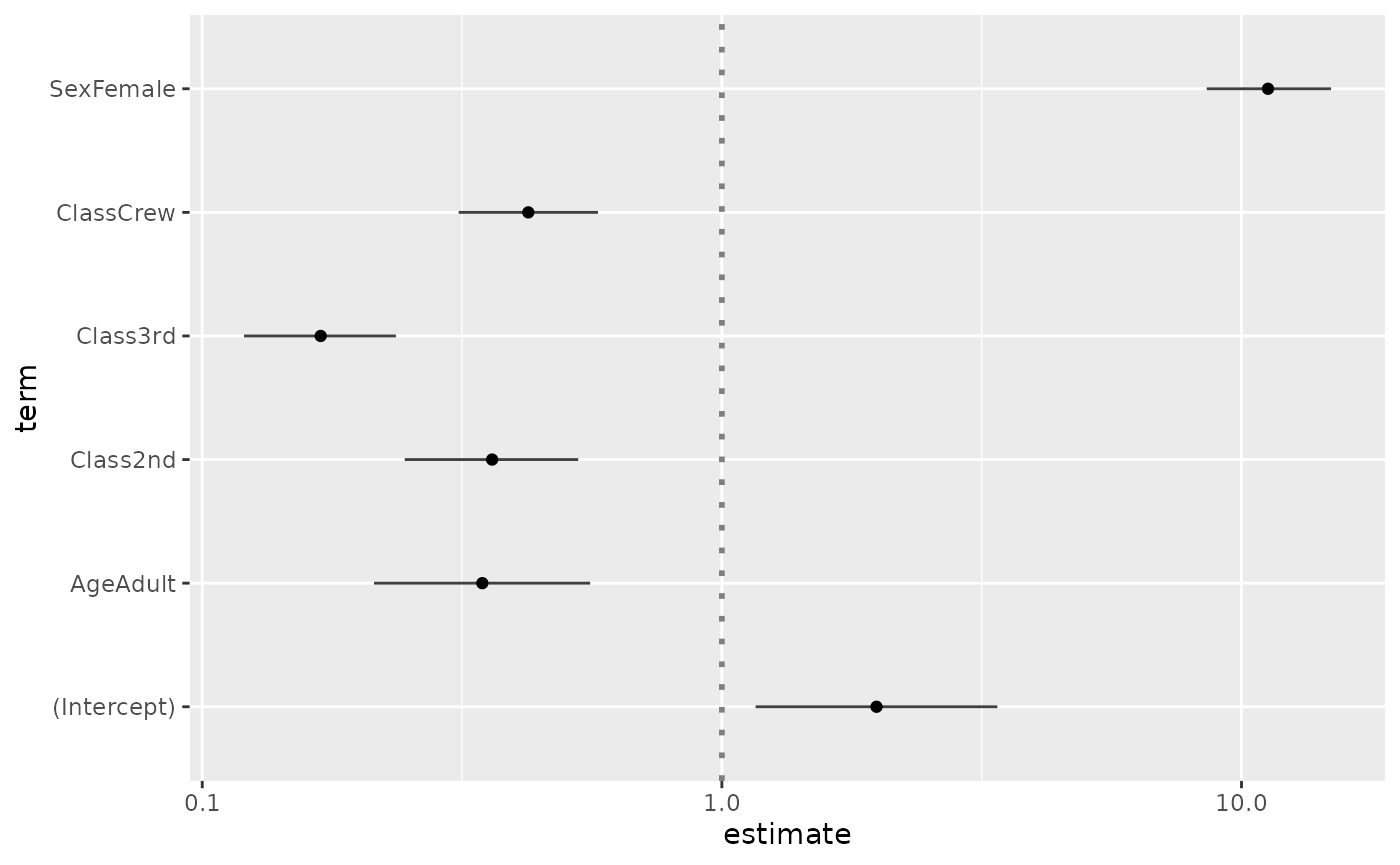## Customizing the plot You can use conf.int, vline and exclude_intercept to display or not confidence intervals as error bars, a vertical line for x = 0 (or x = 1 if coefficients are exponentiated) and the intercept. ggcoef(reg, vline = FALSE, conf.int = FALSE, exclude_intercept = TRUE)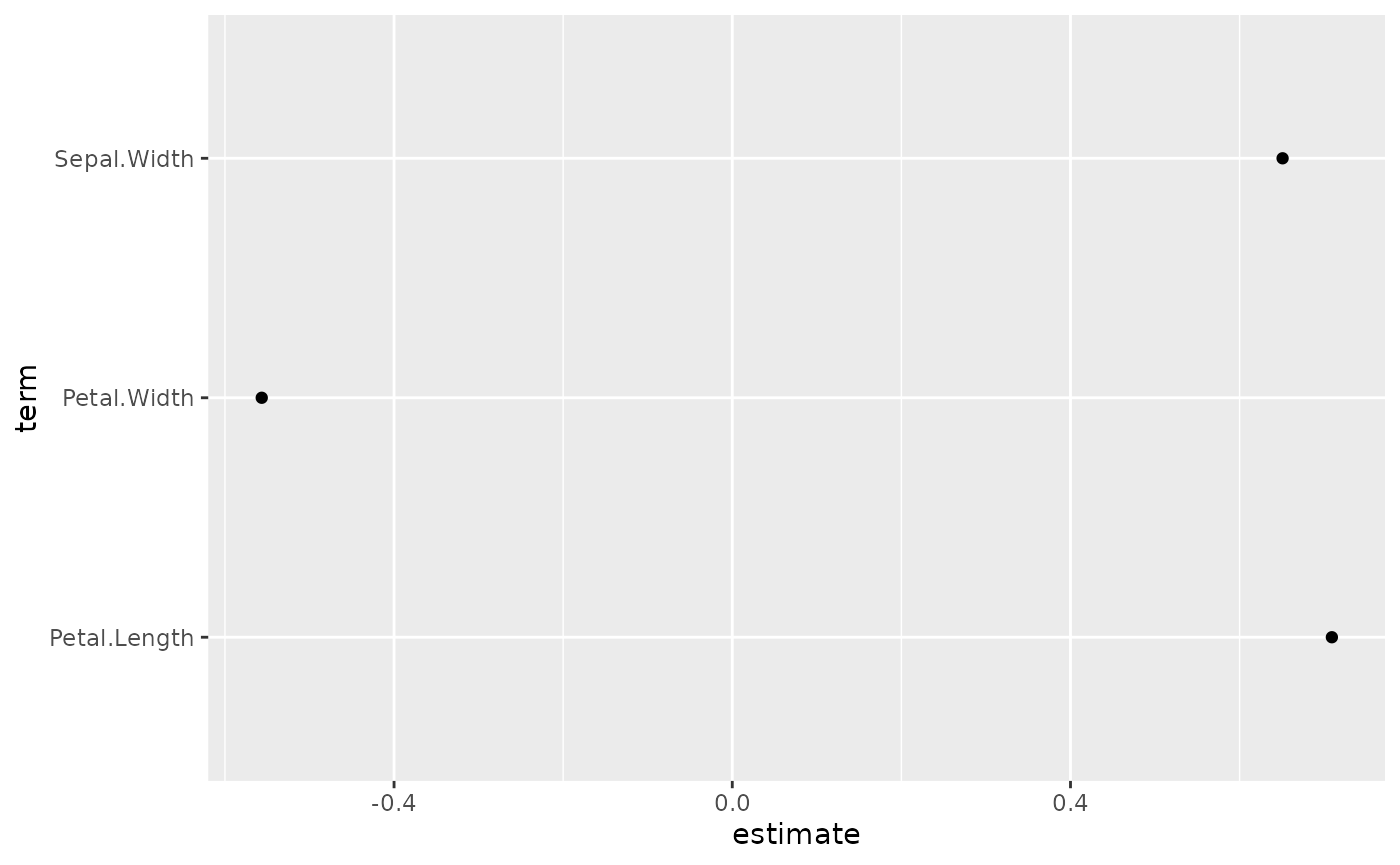See the help page of ggcoef() for the full list of arguments that could be used to personalize how error bars and the vertical line are plotted. ggcoef( log.reg, exponentiate = TRUE, vline_color = "red", vline_linetype = "solid", errorbar_color = "blue", errorbar_height = .25 )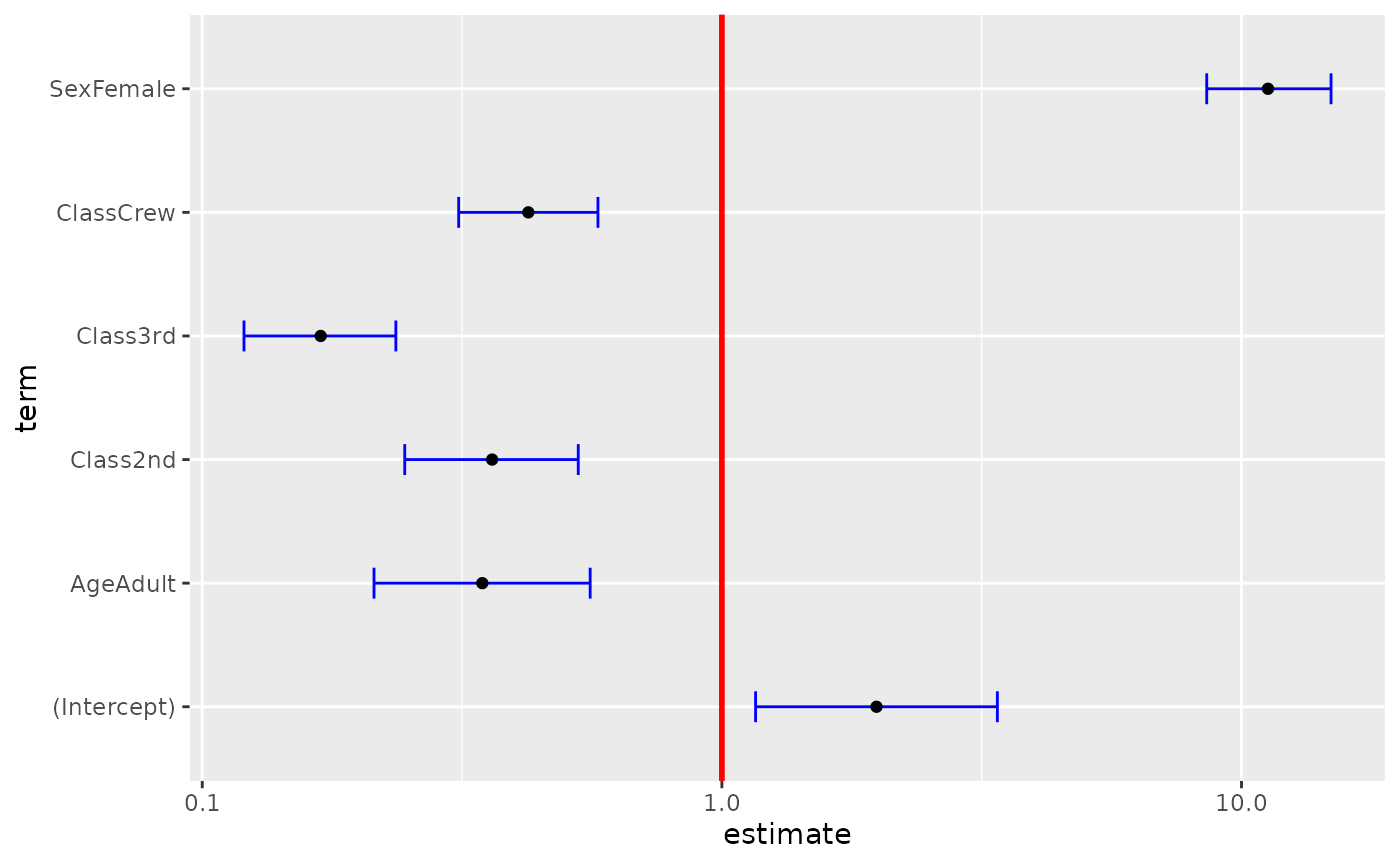Additional parameters will be passed to [ggplot2::geom_point()]. ggcoef(log.reg, exponentiate = TRUE, color = "purple", size = 5, shape = 18)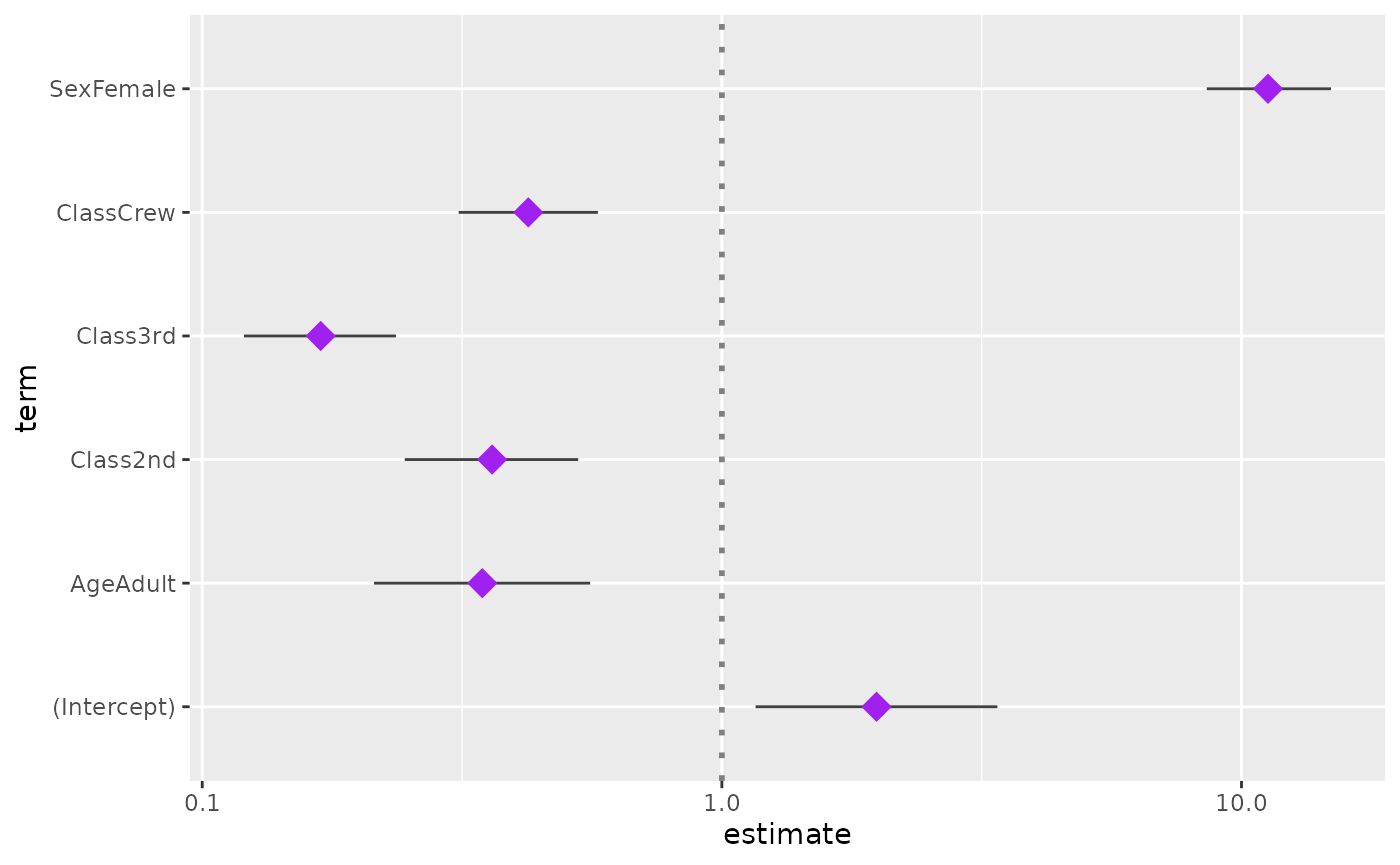Finally, you can also customize the aesthetic mapping of the points. library(ggplot2) ggcoef(log.reg, exponentiate = TRUE, mapping = aes(x = estimate, y = term, size = p.value)) + scale_size_continuous(trans = "reverse")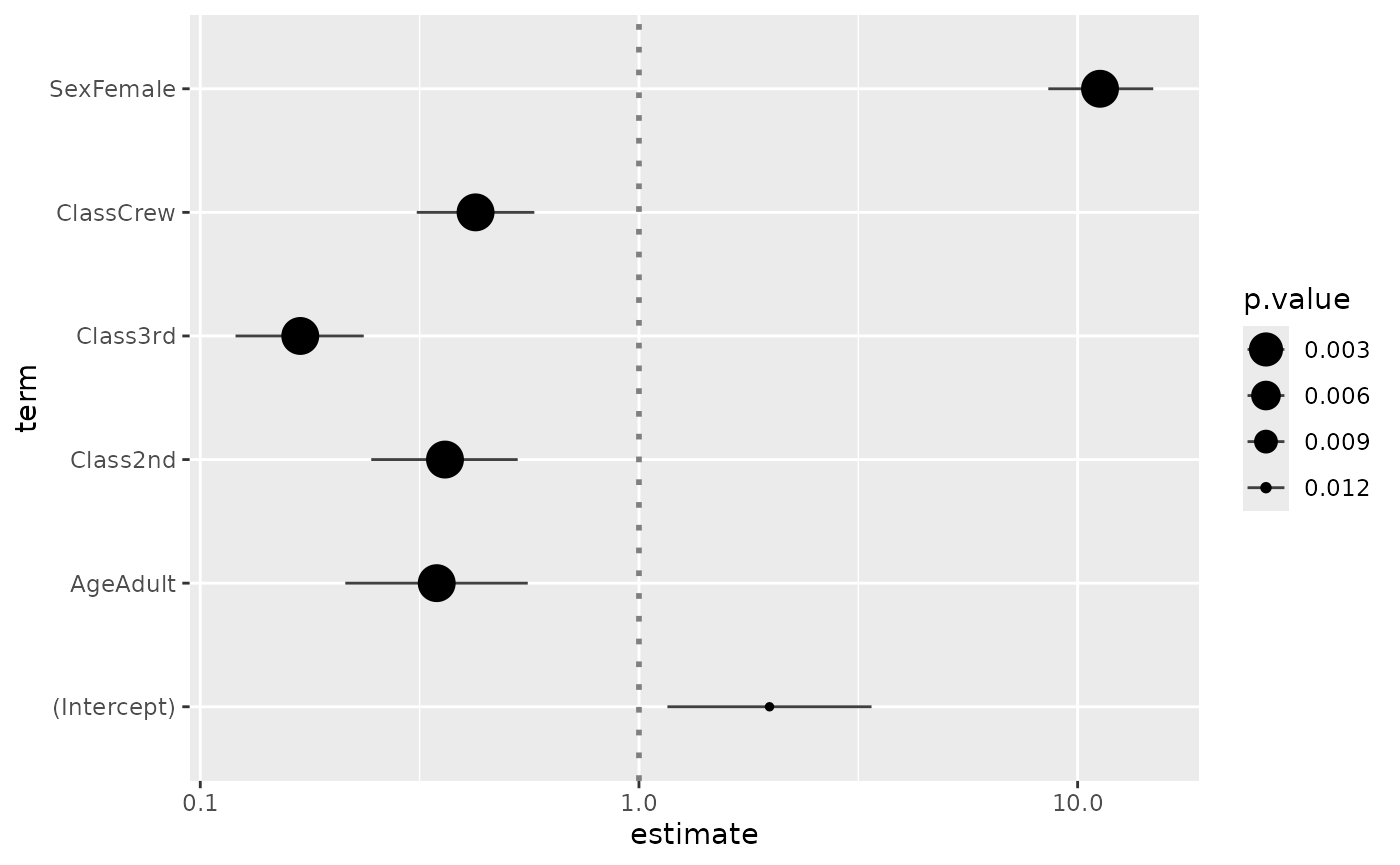## Custom data frame You can also pass a custom data frame to [ggcoef()]. The following variables are expected: • term (except if you customize the mapping) • estimate (except if you customize the mapping) • conf.low and conf.high (only if you want to display error bars) cust <- data.frame( term = c("male vs. female", "30-49 vs. 18-29", "50+ vs. 18-29", "urban vs. rural"), estimate = c(.456, 1.234, 1.897, 1.003), conf.low = c(.411, 1.042, 1.765, 0.678), conf.high = c(.498, 1.564, 2.034, 1.476), variable = c("sex", "age", "age", "residence") ) cust$term <- factor(cust$term, cust$term)
ggcoef(cust, exponentiate = TRUE)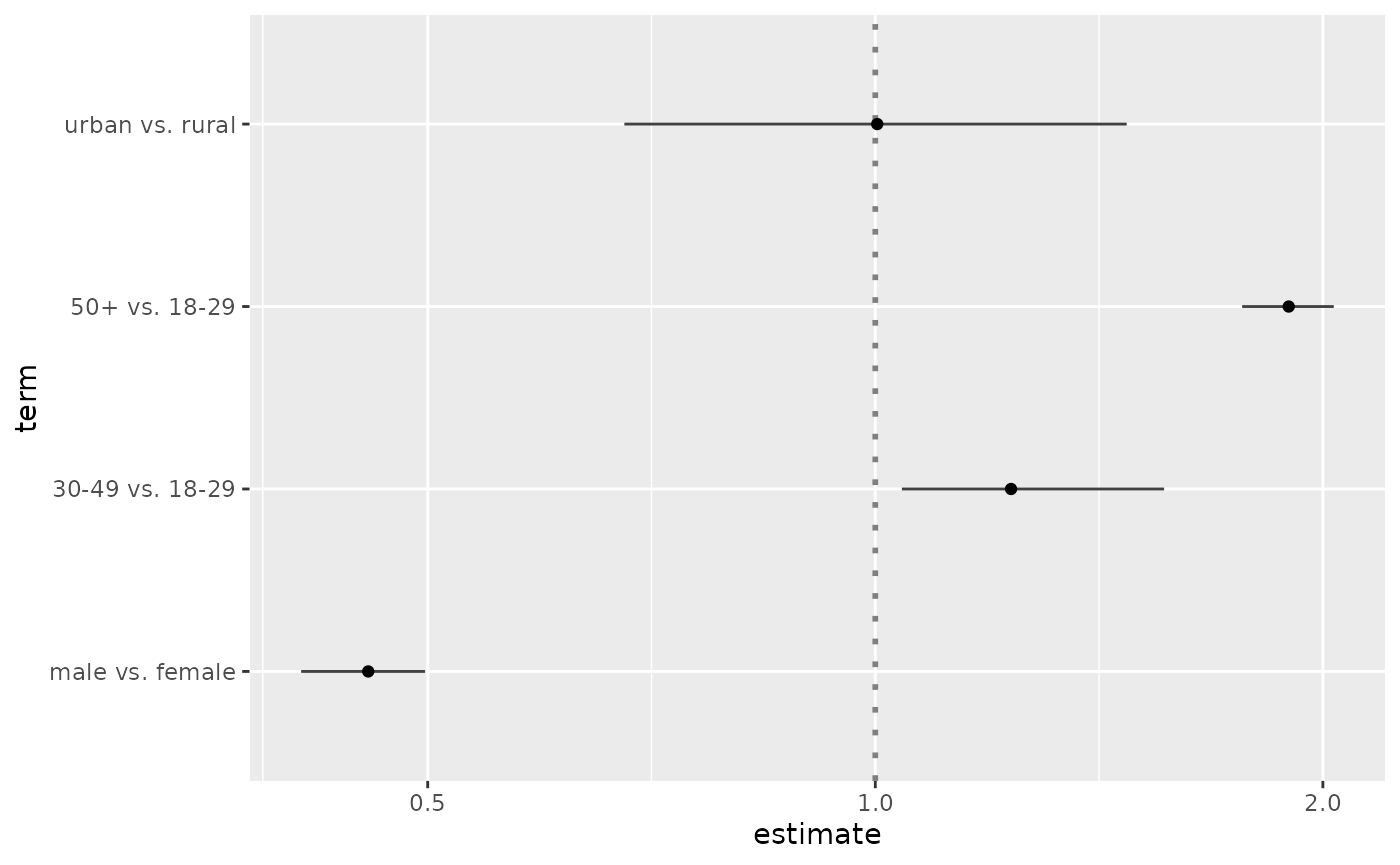ggcoef(
cust,
exponentiate = TRUE,
mapping = aes(x = estimate, y = term, colour = variable),
size = 5
)